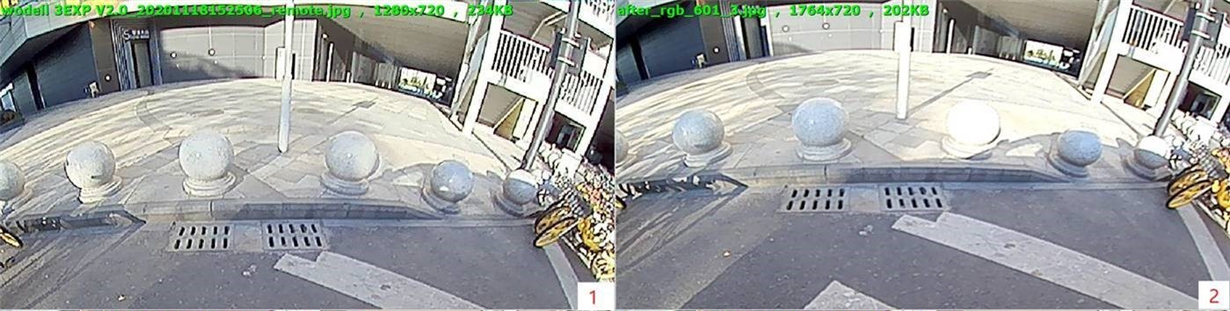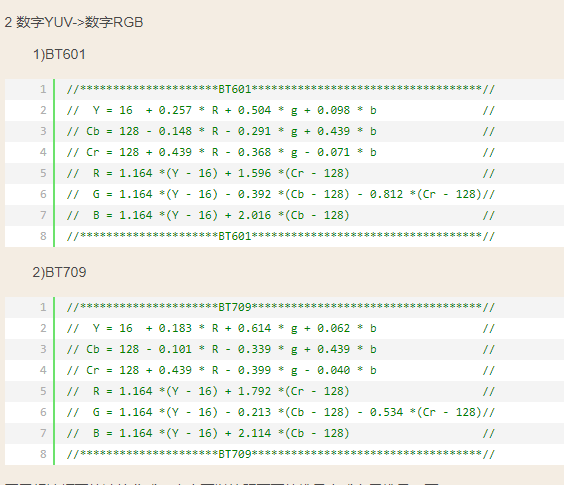If you have a related question, please click the "Ask a related question" button in the top right corner. The newly created question will be automatically linked to this question.

• Resolved

# TDA2HG: How to configure OpenGL YUV2RGB coef matrix

Part Number: TDA2HG

Hi,

We use libEGL to convert YUV420 to RGB888, then we found it overexposed:No.1 picture is captured from camera and No.2 picture is caputred after YUV2RGB.

So we need configure YUV2RGB coef matrix to make it better. Would you please tell me how to configure OpenGL YUV2RGB coef matrix?

Thanks!

• We use libEGL.so to convert YUV2RGB. And my code below:

```  EGLint attr;
int attrIdx;
attrIdx = 0;

attr[attrIdx++] = EGL_LINUX_DRM_FOURCC_EXT;
attr[attrIdx++] = FOURCC_STR("NV12");

attr[attrIdx++] = EGL_WIDTH;
attr[attrIdx++] = width;

attr[attrIdx++] = EGL_HEIGHT;
attr[attrIdx++] = height;

attr[attrIdx++] = EGL_DMA_BUF_PLANE0_PITCH_EXT;
attr[attrIdx++] = width;

attr[attrIdx++] = EGL_DMA_BUF_PLANE1_PITCH_EXT;
attr[attrIdx++] = width;

attr[attrIdx++] = EGL_DMA_BUF_PLANE0_OFFSET_EXT;
attr[attrIdx++] = 0;

attr[attrIdx++] = EGL_DMA_BUF_PLANE1_OFFSET_EXT;
attr[attrIdx++] = 0;

attr[attrIdx++] = EGL_DMA_BUF_PLANE0_FD_EXT;
attr[attrIdx++] = dmaBufFd;

attr[attrIdx++] = EGL_DMA_BUF_PLANE1_FD_EXT;
attr[attrIdx++] = dmaBufFd;

attr[attrIdx++] = EGL_YUV_COLOR_SPACE_HINT_EXT;
attr[attrIdx++] = EGL_ITU_REC601_EXT;                 // set convert as BT601

attr[attrIdx++] = EGL_NONE;

EGLImageKHR textureImg = eglCreateImageKHR(
NativeEGLDisplay,
EGL_NO_CONTEXT,
EGL_LINUX_DMA_BUF_EXT,
NULL,
attr);```

Hi Tim,

A part of the team that can help with this is currently out of office.

We will try to prioritize this early next week. Thanks for your understanding.

Regards

Karthik

• In reply to Karthik Ramanan:

Thanks Karthik.

Dear Customer.

Can we get more details on the use case? you should be able to use a shader to use any coefficients they want.

thank you!

yong

• In reply to Yong Zhang:

Dear Customer,

one more question, Do you want to change the coefficients for just one case or for every conversion?

thank you!

yong

• In reply to Yong Zhang:

Hi yong,

We want change the coefficients for just one case. And now we will trying to use a shader and set coefficients as you suggest. Any update I will let you know.

Thanks!

BR/Tim

• Hi yong,

we have tried use shader to do YUV NV12 to RGB conversion but still found the same overexpose problem.

the coefficients we use:```precision mediump float;

uniform sampler2D  fsSampler;   // Y plane
uniform sampler2D  fsSampler1;  // UV plane

varying vec2 vTexcoord;

vec3 yuv2rgb(vec3 yuv)
{
float r, g, b;

#define DIGITAL_YUV_601
#ifdef DIGITAL_YUV_601
const float rangeY = 16.0 / 255.0;
const float rangeU = 128.0 / 255.0;
const float rangeV = 128.0 / 255.0;
const vec3 yuv2r = vec3(1.164, 0.0, 1.596);
const vec3 yuv2g = vec3(1.164, -0.392, -0.812);
const vec3 yuv2b = vec3(1.164, 2.016, 0.0);
const vec3 range = vec3(rangeY, rangeU, rangeV);
#else  // Analog
const vec3 yuv2r = vec3(1.0, 0.0, 1.402);
const vec3 yuv2g = vec3(1.0, -0.344, -0.792);
const vec3 yuv2b = vec3(1.0, 1.772, 0.0);
const vec3 range = vec3(0.0, 0.0, 0.0);
#endif

yuv = yuv - range;
r = dot(yuv, yuv2r);
g = dot(yuv, yuv2g);
b = dot(yuv, yuv2b);
return vec3(r, g, b);
}

void main (void)
{
vec3 yuv = vec3(texture2D(fsSampler, vTexcoord).r, // Y
texture2D(fsSampler1, vTexcoord).r, // U
texture2D(fsSampler1, vTexcoord).a); // V
gl_FragColor = vec4(yuv2rgb(yuv), 1.0);
}```

Thanks!

• In reply to Wei Xu1:

hi Wei.

would you please show the result when you said "still found the same overexpose problem"? thank you!

yong

• In reply to Wei Xu1:

Hello,

As discussed, there are two ways here:

1. Using GPU for color space conversion and uploading YUV textures using EGL Image (your initial code). With this, you can try FULL range and BT 601/BT 709

attr[attrIdx++] = EGL_YUV_SAMPLE_RANGE_HINT_EXT;

attr[attrIdx++] = EGL_YUV_FULL_RANGE_EXT;   // or EGL_YUV_NARROW_RANGE_EXT – this is default

and

attr[attrIdx++] = EGL_YUV_COLOR_SPACE_HINT_EXT;

attr[attrIdx++] = EGL_ITU_REC709_EXT;

This is completely in the control of the application code. You can try using high precision float.

Is the output different from another GPU?

Regards

Hemant